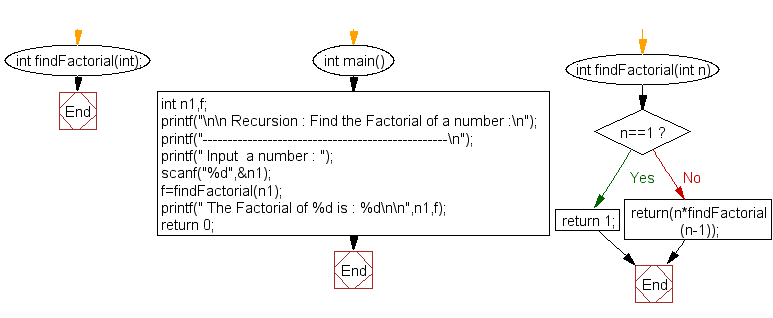﻿ C Program: Find the Factorial of a number - w3resource

# C Exercises: Find the Factorial of a number

## C Recursion : Exercise-10 with Solution

Write a program in C to find the Factorial of a number using recursion.

Pictorial Presentation:Sample Solution:

C Code:

``````#include<stdio.h>
int findFactorial(int);

int main()
{
int n1,f;
printf("\n\n Recursion : Find the Factorial of a number :\n");
printf("-------------------------------------------------\n");
printf(" Input  a number : ");
scanf("%d",&n1);
f=findFactorial(n1);//call the function findFactorial for factorial
printf(" The Factorial of %d is : %d\n\n",n1,f);
return 0;
}

int findFactorial(int n)
{
if(n==1)
return 1;
else
return(n*findFactorial(n-1));// calling the function findFactorial to itself recursively
}
```
```

Sample Output:

``` Recursion : Find the Factorial of a number :
-------------------------------------------------
Input  a number : 5
The Factorial of 5 is : 120
```

Explanation:

```int findFactorial(int n)
{
if(n==1)
return 1;
else
return(n*findFactorial(n-1));// calling the function findFactorial to itself recursively
}
```

The function findFactorial() takes an integer parameter 'n' and returns an integer as the factorial of that number.

The function first checks if 'n' is equal to 1. If it is, then the function returns 1, which is the base case of the recursion. Otherwise, the function recursively calls itself with a parameter of 'n-1' and multiplies the result with 'n'. This continues until the base case is reached (i.e., n=1), at which point the function starts to return the product of 'n' and the result of the recursive call for 'n-1'.

Time complexity and space complexity:

The time complexity of this function is O(n) because the function needs to recursively call itself 'n' times to calculate the factorial of 'n'.

The space complexity is also O(n) because the function call stack will contain 'n' function calls at its peak.

Flowchart:C Programming Code Editor:

Have another way to solve this solution? Contribute your code (and comments) through Disqus.

﻿

## C Programming: Tips of the Day

__FILE__ macro shows full path

```#include <string.h>

#define __FILENAME__ (strrchr(__FILE__, '/') ? strrchr(__FILE__, '/') + 1 : __FILE__)
For Windows use '\\' instead of '/'.
```

Ref : https://bit.ly/3iEWRoT

We are closing our Disqus commenting system for some maintenanace issues. You may write to us at reach[at]yahoo[dot]com or visit us at Facebook

Test your Programming skills with w3resource's quiz.

﻿

## C Programming: Tips of the Day

__FILE__ macro shows full path

```#include <string.h>

#define __FILENAME__ (strrchr(__FILE__, '/') ? strrchr(__FILE__, '/') + 1 : __FILE__)
For Windows use '\\' instead of '/'.
```

Ref : https://bit.ly/3iEWRoT

We are closing our Disqus commenting system for some maintenanace issues. You may write to us at reach[at]yahoo[dot]com or visit us at Facebook